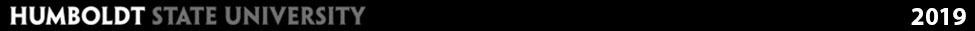# Interaction of EMR with Earth's Surfaces

## Introduction

When electromagnetic energy reaches the Earth's surface there are three possible energy interactions with the surface feature:

• Reflection: occurs when radiation "bounces" off the target and is redirected
• Absorption: occurs when radiation (energy) is absorbed into the target
• Transmission: occurs when radiation passes through a target

As we know from the principle of energy conservation, energy can neither be created nor destroyed, but it can be transferred:EI(λ) = ER(λ) + EA(λ) + ET(λ)
Where:
EI(λ) = Incident energy (from sun)
ER(λ) = Reflected energy
EA(λ) = Absorbed energy
ET(λ) = Transmitted energy

The proportion of energy reflected, absorbed and transmitted will vary depending on the surface material, condition and wavelength of the energy. For example: Vegetation and soils can reflect approximately 30-50% of the incident energy (across the entire EM spectrum) while water on the other hand reflects only 10% of incident energy. Water reflects most of this in the visible range, minimal in the NIR and beyond 1.2 μm (mid-infrared) water absorbs nearly all energy.

## Reflectance

Reflection occurs when incoming energy bounces off a surface and is reflected back. The amount of reflection varies with:

• Wavelength of Energy
• Geometry of the Surface
• Surface Materials
The color of an object is actually the wavelengths of the light reflected while all other wavelengths are absorbed.

### Geometry

The surface geometry of an object is an important consideration when discussing reflectance. This is primarily a function of the surface roughness of an object.

#### Specular Reflectors

Specular reflectors are flat surfaces that produce mirror like reflections. Mirrors and calm surfaces of lakes are specular reflectors and produce mirror-like reflections. On an ideal specular reflector the angle of incidence is equal to the angle of reflection.#### Diffuse or Lambertian Reflectors

Diffuse or Lambertian reflectors are rough surfaces that reflect uniformly in all directions. Most surfaces are idealized diffuse or lambertian reflectors.Most of the surfaces on earth are neither perfectly specular or diffuse reflector but their characteristics are somewhere in between.The total quantity of incoming energy (light) from the sun is known as irradiance. Satellites measure radiance (brightness), or the amount of light. Objects on the ground are often characterized by their reflectance, or the percentage of the total energy that is reflected. The atmosphere affects the radiance received by the sensor in two ways:

1. It can reduce (or attenuates) the energy
2. Atmosphere itself is a reflector, adding energy or “path radiance” to the signal detected by the sensor

In remote sensing we are generally interested in the reflectance characteristics of surface features. Reflectance is the percent of incoming incident energy that is reflected. This is always measured as a function of wavelength and is given as a percent.

Spectral Reflectance
ρλ = ER(λ) / EI(λ)
or
% Reflectance = Amount of Reflected Energy/Total Energy x 100

← Back

Next →

Module Home